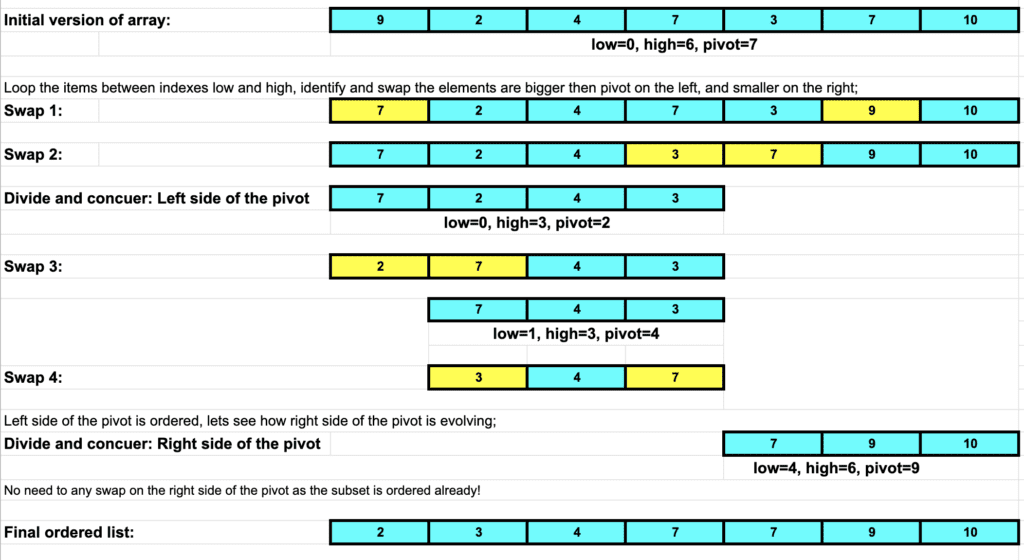# Technical Interview Questions: Understanding Quicksort AlgorithmThe third round of interview questions wouldn’t be fair if we didn’t mention a sorting algorithm! So, in this tutorial, I decided to go through one of the most efficient sorting algorithms: The Quicksort Algorithm!

## What is the Quicksort Algorithm?

Quicksort is one of the most efficient sorting algorithms and an excellent question for an interview.

The best-case time complexity of Quicksort is O(n log n), average-case complexity is O(n log n), and O(n^2) in the worst case. So, of course, the dataset plays a significant role in the complexity of any sorting algorithm. But there is no harm in mentioning that Quicksort is considered the fastest sorting algorithm most of the time.

## How Does Quicksort work?

The Quicksort algorithm works on the principle of repeatedly dividing and conquering. It always picks an element as a pivot, splits the array of data into smaller chunks, sorts sub-arrays, and then combines them.

Three steps of Quicksort;

Choose the pivot:

Quicksort algorithm has different implementations for pivot selection, but the aim is to choose the middle element as the pivot. Various algorithms determine the first or last item as a pivot.

Divide and conquer the data:

Based on the chosen pivot pointer, the array of items smaller than the pivot will be placed on the left side, and the array of items bigger than the pivot will be placed on the right side of the pivot.

Combine (if needed):

Repeat the steps until all data is sorted, and then combine the sorted sub-arrays.

## Quicksort Implementation in Java

The below implementation is from programcreek.com and uses the middle element of the array as a pivot and orders based on that.

```				```
public static void quickSort(int[] arr, int low, int high) {
if (arr == null || arr.length == 0)
return;

if (low >= high)
return;

// pick the pivot
int middle = low + (high - low) / 2;
int pivot = arr[middle];

// make left < pivot and right > pivot
int i = low, j = high;
while (i <= j) {
while (arr[i] < pivot) {
i++;
}

while (arr[j] > pivot) {
j--;
}

if (i <= j) {
int temp = arr[i];
arr[i] = arr[j];
arr[j] = temp;
i++;
j--;
}
}

// recursively sort two sub parts
if (low < j)
quickSort(arr, low, j);

if (high > i)
quickSort(arr, i, high);
}
```
```

Quicksort Algorithm Iteration for a pre-defined dataset;## Conclusion

Quicksort algorithm gives instructions about sorting a dataset in a few steps but does not restrict you with the step details. There might be different implementations for pivot selection and different swap algorithms, and even the way you combine data might be different.  In that sense feel free to check out different implementations of Quicksort.

Exceptionly Tech Blog is a great source to keep yourself up to date as it challenges tools, best practices, and methodologies.